The equations of the asymptotesCustom SearchThe equations of the asymptotes were given earlier as bx+ay=0 and bx-ay=0 or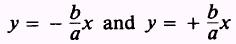The earlier reference also pointed out that the length of the focal chord is equal to. Note that you have no restriction of a > b for the hyperbola as you have for the ellipse. Instead, the direction in which the hyperbola opens corresponds to the transverse axis on which the foci and vertices lie. The properties of a hyperbola can be determined from the equation of a hyperbola or the equation can be written given certain properties, as shown in the following examples. In these examples and in the practice problems immediately following, all of the hyperbolas considered have their centers at the origin. EXAMPLE: Find the equation of the hyperbola with an eccentricity of 3/2, directrices x = ± 4/3, and foci at ( ± 3,0). SOLUTION: The foci lie on the X axis at the points (3,0) and ( - 3,0), so the equation is of the formThis fact is also shown by the equation of the directrices. Since we have determined the form of the equation and since the center of the curve in this section is restricted to the origin, the problem is reduced to finding the values of a2 and b2. First, the foci are given as (± 3,0); and since the foci are also the points (± c,0), then c=3 The eccentricity is given and the value of a2 can be determined from the formula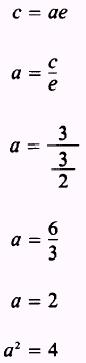The relationship of a, b, and c for the hyperbola is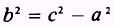andWhen these values are substituted in the equation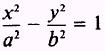the equation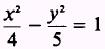results and is the equation of the hyperbola. The equation could also be found by the use of other relationships using the given information. The directrices are given as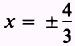and, sinceor a=de substituting the values given for d and e results in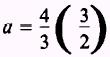therefore, a=2 and a2 = 4 While the value of c can be determined by the given information in this problem, it could also be computed since c = ae and a has been found to equal 2 and e is given as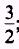; therefore,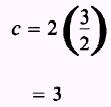With values for a and c computed, the value of b is found as before and the equation can be written. EXAMPLE: Find the foci, directrices, eccentricity, length of the focal chord, and equations of the asymptotes of the hyperbola described by the equationSOLUTION: This equation is of the form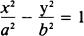and the values for a and b are determined by inspection to beandWith a and b known, we find c by using the formula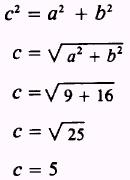From the form of the equation, we know that the foci are at the pointsand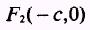so the foci =The eccentricity is found by the formula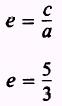Figure 2-17 shows that with the center at the origin, c and a will have the same sign. The directrix is found by the formulaor, since this equation will have directrices parallel to the Y axis, by the formulaThenSo the directrices are the lines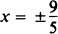The focal chord (f .c.) is found byFinally, the equations of the asymptotes are the equations of the two straight lines: bx+ay=0 and bx-ay=0 In this problem, substituting the values of a and b in each equation gives 4x+3y=0 and 4x-3y=0 or 4x±3y=0 The equations of the lines asymptotic to the curve can also be written in the formandIn this form the lines are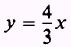and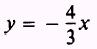or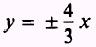If we think of this equation as a form of the slope-intercept formula y=mx+b from chapter 1, the lines would have slopes of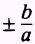and each would have its y intercept at the origin as shown in figure 2-18.Integrated Publishing, Inc. - A (SDVOSB) Service Disabled Veteran Owned Small Business